## research papersJOURNAL OFSYNCHROTRONRADIATION
ISSN: 1600-5775
Volume 25| Part 5| September 2018| Pages 1346-1353

## Characterization and on-line adjustment of the sagittal-bent Laue crystal profile

aBeijing Synchrotron Radiation Facility, Institute of High Energy Physics, Chinese Academy of Sciences, 19B Yuquan Road, Shijingshan District, Beijing 100049, People's Republic of China, and bSchool of Physical Sciences, University of Chinese Academy of Sciences, 19A Yuquan Road, Shijingshan District, Beijing 100049, People's Republic of China
*Correspondence e-mail: hulf@ihep.ac.cn

(Received 3 February 2018; accepted 31 May 2018; online 2 August 2018)

The sagittal-bent Laue monochromator can provide an ideal way to focus high-energy X-ray beams. However, the anticlastic curvature induced by sagittal bending has a great influence on the crystal performance. Thus, characterizing the bent-crystal shape is very important for predicting the performance of the bent-crystal monochromator. In this paper the crystal profile is measured by off-line optical metrology and on-line X-ray experiments. The off-line results showed that the bent-crystal surface could be well fitted to a saddle surface apart from a redundant cubic term which was related to the different couples applied on the crystal. On-line characterization of the meridional and the sagittal radius of the bent crystal includes double-crystal topography and ray-tracing measurement. In addition, the double-crystal topography experiment could be used as a quick diagnostic method for the bending condition adjustment. The sagittal radius of the bent crystal was characterized through a ray-tracing experiment by using a particularly designed tungsten mask. Moreover, rocking curves under different bending conditions were measured as well. The results were highly consistent with analytical results derived from the elastic theory. Furthermore, radii along different vertical positions under various bending conditions were measured and showed a quadratic relationship between the vertical positions and the meridional radii.

### 1. Introduction

The sagittal-bent double-Laue crystal monochromator was proposed by Zhong et al. (2001a,b) to focus high-energy (>30 keV) X-ray beams in the horizontal direction, especially for wiggler and bending-magnet sources with wide horizontal incident area. It is well known that, owing to the elastic property of single Si crystal, sagittal bend can induce anti­clastic bending in the meridional plane (Chukhovskii et al., 1994; Timoshenko & Goodier, 1969). Furthermore, because of the changing orientation and the spacing of lattice planes caused by bending, the width of the rocking curve is broadened (Albertini et al., 1976; Suortti et al., 1992) by about one order of magnitude. Thus, taking the anisotropic mechanical property of single-silicon crystal into consideration, by choosing appropriate lattice plane, crystal thickness and asymmetry angle (angle between the reflection plane and the surface normal) it is possible to obtain the optimal rocking-curve width. Rather than restraining the anticlastic bending in other diffraction geometries (Koyama et al., 1992; Pascarelli et al., 1996; Feng et al., 2008; Nisawa et al., 2013), sagittal-bent Laue crystals utilize the anticlastic bending to match the Rowland circle in the meridional plane. In doing so, it is possible to take advantage of the wider angular acceptance and the higher photon flux with less reduction in the energy resolution.

Crystal-profile characterization consists of measuring two radii of curvatures corresponding to orthogonal directions. This can be done off-line by optical metrology such as by using a long trace profiler (LTP) or interferometer (Feng et al., 2008; Shi et al., 2011). However, in practice one would like to change the bending curvature to achieve different focusing conditions. This requires on-line feedback on the adjustment. In this paper, we present the measurement of anticlastic curvature using double-crystal X-ray topography (Jacobs & Hart, 1977; Bowen & Tanner, 1998). This experiment was conducted at the 4W1A beamline of Beijing Synchrotron Radiation Facility (BSRF). White beam was delivered to the experiment hutch. A channel-cut crystal was used to obtain a 20 keV monochromatic beam. An asymmetrically cut Si (220) crystal was used to expand the incident X-ray beam to the tested crystal. Fig. 1(a) gives an illustration of the double-crystal topography experiment setup after the monochromatic beam. As bending induced the lattice-plane-orientation variation, only a small portion of the crystal slab satisfies Bragg's condition when exposed to large X-ray beams, thus only one small stripe appears on the CCD. By rocking the bent crystal, a series of stripes appear successively on the CCD plane and merge to a known `zebra stripes' pattern (Jacobs & Hart, 1977; Martinson et al., 2017). The pattern of zebra stripes provides a way to monitor the crystal profile uniformity during the adjustment. Typical zebra stripe patterns are illustrated in Fig. 1(b). Through analysing the rocking angles and the corresponding stripe intervals, we can calculate the anticlastic radius of curvature. To obtain the sagittal-bending information, we used a ray-tracing measurement in the horizontal direction. Considering the limited space of the 4W1A experiment hutch, this experiment was conducted at the BL09B beamline of Shanghai Synchrotron Radiation Facility (SSRF). These studies provide a rapid feedback on the on-line adjustment of the bending conditions.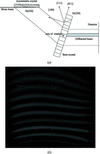Figure 1 (a) Schematic of the double-crystal topography measurement. The asymmetric crystal is Si (220) with an asymmetry angle of 82° which results in a magnification factor of 13.1. The measured lattice plane of the tested bent crystal is also (220). (b) A typical zebra stripe pattern produced by merging stripes at different pitch angles. Each stripe indicates the satisfaction of Bragg's law. The adjacent stripes correspond to a change of 5 arcsec in diffraction angles.

### 2. Mechanical design of the sagittal bender

The bender is designed according to the four-roller fixed-height exit bending mechanism (Yoneda et al., 2001). Two rotation arms are set symmetrically. On each arm two rollers are mounted for applying torque to the crystal. The distance between the rotation axis (OA and OB in Fig. 2a) and the centre of the crystal is 20 mm, which is equal to the distance from the rotation axis to the midpoint of the two rollers (E and F in Fig. 2a) on the same side. This design ensures a fixed centre for the bent crystal when changing its bending radius. Fig. 2(b) shows an engineering drawing of the instrument. The benders were equipped with two picomotors (New FocusTM, 8322NF), one for changing the bending radius and the other for adjusting the sample crystal twist. By adjusting the bend motor, the arms rotate around the axes oppositely to change the bending radius. The twist modification is achieved through adjusting the parallelism of the two roller pairs. The twist motor is mounted on one arm so that altering the bending radius will not influence sample distortion.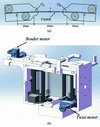Figure 2 (a) Schematic of the bending mechanism when the crystal is bent. OA and OB are the rotation axes of two arms, E and F are on the midpoint of two pairs of rollers. By setting OAOB = 2OAE = 2OBF, the centre of the crystal can be approximately fixed when changing bending radii. (b) Assembly engineering drawing of the sagittal bender.

As shown in Fig. 1(a), the Laue crystal is rectangular in shape and was cut from a polished silicon (100) wafer with a size of 110 mm × 70 mm and a thickness of 0.67 mm. The long side is parallel to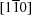and the short side is parallel to   . In this experiment the symmetric (220) diffraction plane and the asymmetric (111) diffraction plane with an asymmetry angleχ = 35.3° were used.

### 3. Off-line surface measurement

The fairly large difference between the sagittal radius and the meridional radius makes it impossible to adopt commercial interferometer products to measure the bent-crystal surface profile directly. To solve this problem, we have developed two different techniques. One is the wavefront-matching technique and the other is the angle-stitching technique. The wavefront-matching technique is used to match the tested surface and the reference parabolic cylinder wavefront. The parabolic cylinder wavefront is produced by inserting a cylindrical lens into the interferometer optical path. On the other hand, the angle-stitching technique is used to stitch the sparse areas of the interference fringes gathered from different tilt angles in the sagittal direction. A self-collimator (Möller-WEDEL OPTICAL GmbH, Elcomat 3000) is used for high-precision angle measurement. By using the appropriate algorithm one can derive the tested bent crystal surface. A Zygo Verifire QPZ interferometer was used. A large number of experiments have proven that both techniques can achieve high-precision surface measurement. In this paper we show results of the angle-stitching technique.

After filtering the high-frequency roughness, which is of little concern to this application, the measured surface fits well to a saddle surface (Fig. 3). As illustrated in Fig. 3, the main part of the measured surface is a hyperbolic paraboloid (saddle surface) with the fitted centre of the surface at xc = 15.68 mm and yc = 4.96 mm. The radii of curvature around the centre point are Ry = 40.23 m in the y direction and Rx = 1.69 m in the x direction, corresponding to the meridian radius Rm and the sagittal radius Rs of the sample crystal, respectively. In addition, the surface total translation f is negligibly small, of the order of 10−4 mm, and the coefficient c of the xy term is 2.72 mm−1 which shows a negligible x–y coordinate axis rotation (see Appendix A). Thus, the main hyperbolic-paraboloid surface can be written as z = (yyc)2/2Ry − (x − xc)2 / 2Rx + c(xxc)(yyc) + f. It is interesting to point out that after subtracting the saddle surface a regular cubic surface z = a(xxc)3 with a = 1.30 m−2 remains. This can be explained by the unequal couples applied on each end of the crystal slab. If we treat the crystal slab as a beam, which is a fair assumption to the first order, the system could be treated under the framework of the Euler–Bernoulli beam theory (Young & Budynas, 2002). Because of the different couples on each end, the shape of the beam can be expressed as (Sutter et al., 2012, 2017)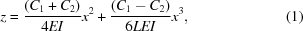where C1 and C2 are the different couples, E is Young's modulus of the crystal, I is the moment of inertia and L is the beam length. The equation above clearly shows that the cubic curve occurs when two couples C1 and C2 are different. Thus, we can infer that the cubic term of the measured surface is caused by the minor difference between the couples applied on each end of the crystal slab.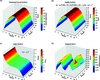Figure 3 (a) Surface profile of the measured bent crystal using a stitching interferometer. The high-spatial-frequency data are filtered. (b) The main part of the fitted hyperbolic-paraboloid surface (saddle surface). The surface equation is z = (y − yc)2 / 2Ry − (x − xc)2 / 2Rx + c(x − xc)(y − yc) + f. (c) The regular cubic term of the fitted measured surface is z = a(x − xc)3. This term can be explained by the unequal couples applied on each end of the crystal slab. (d) The fitting errors of the measured surface.

### 4. Double-crystal topography measurements

#### 4.1. Method and experiment

The beamline geometry is vertical bouncing and a side view of the double-crystal topography experiment setup is illustrated in Fig. 1(a). A highly asymmetrical flat crystal is placed in front of the tested bent crystal. By asymmetrical cut, the incident area is expanded on the bent-crystal surface. If the tested crystal is flat, at Bragg's angle of the lattice plane, the diffracted X-ray beam from the tested crystal will form a large bright area on the detector. After bending, the intrinsic anticlastic deformation gives rise to the lattice-plane-orientation variation. As a result, the diffracted X-ray beam on the detector is the only stripe which satisfies Bragg's law. The stripe on the detector moves with the rocking of the tested crystal. Thus a series of single stripes obtained at different pitch angles merge to a zebra pattern which can be viewed as a strain contour mapping (Fig. 1b). The obtained field of view covered the whole working design area [30 mm (H) × 10 mm (V)] of the bent-crystal monochromator.

The experiment was conducted at 4W1A beamline of BSRF. White beam was delivered to the experiment hutch. Fig. 4gives a side view of the experiment setup. As shown in the figure, the experiment has two modes. The Si (111) channel-cut monochromator provides 20 keV monochromatic beam for each mode. The upper optical path (upper case) is the double-crystal X-ray topography experiment. The asymmetric crystal is Si (220). The asymmetry angle is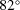with an asymmetry factor of b = 13.1, which supplies 13× magnification of the tested crystal's exposure area. The parameters of the tested bent crystal have been described before. In the double-crystal X-ray topography experiment, the (220) lattice plane of the bent crystal was chosen. Thus, the outgoing beam was horizontal. A CCD with 6.5 µm × 6.5 µm pixel size was used to record the zebra stripe patterns. The lower optical path (lower case) was designed to measure the Si (111) rocking curves under different bending conditions. This case consists of a channel-cut monochromator and the tested bent crystal. A photodiode was used to measure the rocking curves. The asymmetry crystal and the tested bent crystal can be moved up and down by electro-motors when switched from one case to the other.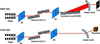Figure 4 Schematic of the experimental setup at 4W1A (BSRF). The upper case shows an enlarged beam path with an asymmetric crystal Si (220), for the measurement of the zebra pattern. The lower case is the setup for the rocking-curves measurement with a pencil beam, where the asymmetric crystal was moved out and the bent crystal was down to the beam height of the channel-cut Si (111).

The anticlastic radius of curvature can be calculated by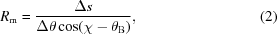where Δs is the stripe interval on the CCD, Δθ is the fixed rocking step, χ is the asymmetry angle of the diffracted lattice plane and θB is Bragg's angle.

#### 4.2. Results and discussions

Fig. 5gives the zebra stripe patterns measured at the upper mode and the rocking curves at the lower mode under the same bending conditions. From Fig. 5(a) we can see that the stripe moves when rotating the tested bent crystal. Therefore, from equation (2)we can calculate that the central radius of curvature in the meridian plane is about 152.73 m. From Fig. 5(b) we find that, owing to the vertical translation of the tested bent crystal, the peaks of the rocking curves varied. From the varied rocking-curve peaks and the fixed vertical-translation steps, we can also use equation (2)to calculate the meridian radius of curvature. Here, Δs in equation (2)is written as Δh, which means that the vertical height difference of the adjacent strips and Δθ is the interval of the rocking-curve peaks. The calculated radius is about 159.11 m. These two measurements are within the accepted error of 5–10% for the meridian radius of curvature Rm determination. Thus, we believe that these two methods could both be applied to the measurement of Rm. Although both methods can measure the radius of curvature in the meridian direction, we have to point out that the zebra stripe patterns provide quick on-line feedback to the adjustment of different bending conditions.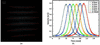Figure 5 (a) Zebra stripes pattern measured at the upper case by rotating the test bent crystal with a fixed angle of Δθ = 12 µrad. (b) Rocking curves measured at the lower case. The peaks of the rocking curves varied when we changed the vertical height of the tested bent crystal by Δh = 2 mm at each time.

When rotating the bent Laue crystal with a fixed angle, the vertical position of the sample which satisfies Bragg's law changes. Fig. 6shows the calculated meridian radii of curvatures with respect to the rotation angles of the bent Laue crystal under different sagittal bending conditions. The radii at different rotation angles can be fitted to a quadratic curve. These results show that the anticlastic radius of curvature has a quadratic relationship with the rotation angles (different vertical positions) rather than a fixed value under real bending conditions.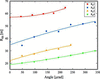Figure 6 The calculated meridian radii of curvature with respect to the rotation angles of the bent Laue crystal under different sagittal bending conditions. Rs1–Rs4 represent four sagittal bending radii. When rotating the crystal, the vertical positions of the sample that satisfy Bragg's law also change.

Furthermore, if we plot the different meridian radii and the corresponding rocking-curve widths (FWHMs) in one figure, we can see a linear relationship between the rocking-curve width and the reciprocal of the anticlastic radius 1/Rm (Fig. 7). This relationship can be explained by the mechanical properties of the bending crystal. The rocking-curve width of a perfect Si (111) plane Laue crystal at 20 keV is 12.85 µrad (ω0). Compared with the measured width (FWHM), ω0 is very small. Therefore, the dominant influence comes from the lattice orientation and lattice-spacing variations. Taking anisotropic mechanical properties of the bent Si crystal into consideration, the total change in Bragg's condition caused by bending is (Zhong et al., 2002; Shi et al., 2011)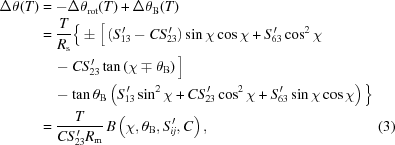where T is the crystal thickness, Rs is the sagittal radius, Rm is the meridian radius, χ is the asymmetry angle, θB is Bragg's angle,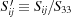, Sij are the elastic compliances of the crystal and C is an empirical parameter in order to give a simple analytical expression for the change in Bragg condition. Rs and Rm are connected by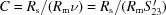. In our measurement ranges, the empirical parameter C is an invariant value. Apart from Rm, all the other parameters in equation (3)are invariable. Thus, we can see from equation (3)that the measured rocking-curve width is proportional to 1/Rm which agrees with Fig. 7.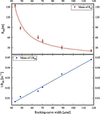Figure 7 Relationship between the meridian radii and the rocking-curve widths (FWHMs) at different bent conditions. Top: relationship between the measured rocking-curve widths and the meridian radii at different bending conditions. The mean of Rm and the rocking-curve widths fitted well in a reciprocal function. Bottom: the rocking-curve width versus the reciprocal of the anticlastic radius 1/Rm, showing a linear relationship between them.

### 5. Ray-tracing characterization of sagittal bending

The ray-tracing experiment was performed at beamline BL09B of SSRF. This beamline is dedicated to X-ray optics tests. The experiment was conducted with a double-Laue monochromator configuration (DLM, see Fig. 8). The sagittal radius of the first crystal was bent to 1.2 m and was measured by optical metrology before being installed in the optical path. The second crystal was dynamically bent. The selected beam energy was 80 keV.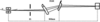Figure 8 Schematic of the double Laue monochromator (DLM) configuration at BL09B (SSRF).

A tungsten mask with equidistant circles was placed at the end of the beamline vacuum pipe. It divided the incident beam into a periodic array of segmented beams in the horizontal direction. A CCD was used to record the DLM reflected beam. Owing to the beam focusing in the horizontal direction, when we placed the CCD at different positions a series of images with a converging trend were obtained. These images allowed us to calculate the focal distance of the DLM using geometric optics principles. Fig. 9shows images of the tungsten mask at various positions. After a series of image processing, we put the circles of each image into one identical coordinate system. By linking the centres of these circles (Fig. 10a), we were able to extrapolate the image distance of the DLM. Fig. 10(b) shows the extrapolation results. The extrapolated image distance is 82 m. For the DLM, the sagittal imaging relation is (Zhong et al., 2001a)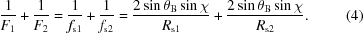Note that Rs1 and Rs2 are the sagittal radii of curvature of the first and second bent crystals, F1 is the distance between the source and the DLM, F2 is the distance between the DLM and the focal point,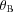is Bragg's angle and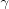is the asymmetry angle. In this experiment the distance between the source and DLM is about 39 m and the distance from the DLM to the extrapolated focal point is about 82 m. The sagittal radius of the first crystal is 1.2 m, Bragg's angle at 80 keV is 1.416° and the asymmetry angles of both crystals are 35.3°. Therefore, using equation (4), we calculated that Rs2 was about 2.0 m. Since the designed sagittal radius (1.2 m) was not obtained, we could adjust the bending condition to obtain a smaller radius. Based on the above description, it is fair to assert that the ray-tracing measurement provides a quick feedback on sagittal-radius adjustment.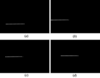Figure 9 Images of the tungsten mask at various distances from the first crystal, 2.4 m (a), 4.1 m (b), 5.5 m (c) and 7.0 m (d).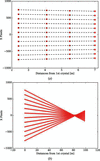Figure 10 (a) The measured centres of the dots when the CCD was placed at different positions from the first crystal. The centres fit well to a straight line which can be viewed as the light ray. (b) The extrapolated result. The image distance of this bending condition is 82 m from the DLM.

### 6. Conclusion

In this paper, various measurement techniques were employed to characterize the bent Laue crystal monochromator. Off-line optical methodology was used to measure the surface of the bending crystal. From the results of these measurements we have proven that the surface can be fitted well to a saddle surface. To measure the meridian radius of the crystal, we used two techniques: double-crystal topography and rocking-curve measurement. These two techniques are consistent with each other. Yet, the double-crystal-topography technique can provide a quick feedback on the on-line adjustment that the rocking-curve measurement could barely achieve. Using these two techniques we measured the relationship between the rocking-curve width and the meridian radius. The result conformed to the simple mechanical model under the condition of small deformation. Furthermore, to characterize the sagittal bending a ray-tracing measurement was carried out. Under the framework of geometrical optics, we were able to extrapolate the image distance of the DLM and then calculate the sagittal radius.

Apart from the optical methodology measurement, the double-crystal topography, rocking-curve and ray-tracing measurements are all on-line methods. This means that these methods can be used to provide quick feedback on the adjustment of the bending condition. Although methods described in this discussion offer quick feedback on the on-line adjustment, achieving higher-accuracy result is the subject of ongoing research.

### Standard form of the fitted quadric surface

A generic quadric surface has the form z = a1x2 + a2y2 + a3xy + a4x + a5y + a6 with six independent coefficients a1, a2, a3, a4, a5 and a6. In this paper, we use the form z = (yyc)2/2Ry − (xxc)2/2Rx + c(xxc)(yyc) + f, which also has six independent coefficients. Thus, these two forms are equivalent. From the forms we used, we can easily figure out that, after translation transformation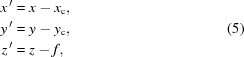the formula of the saddle surface can be written as z = y2/2Ryx2/2Rx + cxy. In matrix form, we have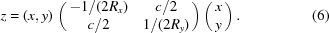Basic linear algebra results show that the real symmetric matrix can be diagonalized by an orthogonal matrix. The 2 × 2 orthogonal matrix can be written as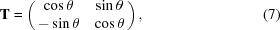and we have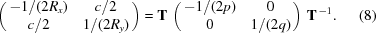This means that, with appropriate orthogonal transformation, the saddle surface can be changed into a standard form of z = −x2/2p + y2/2q. The orthogonal transformation is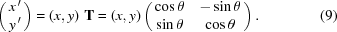This means that the x–y plane rotates by angle θ around the origin of the coordinates. −1/(2p) and 1/(2q) are the eigenvalues of the matrix, and the column vectors of matrix T are the eigenvectors. With the fitted coefficients Ry = 40.23 m, Rx = 1.69 m, c = 2.72 mm−1, the eigenvalues of the corresponding diagonal matrix for z = y2/2Ryx2/2Rx + cxy are −0.296 m−1 and 0.0124 m−1, equivalent to p = 1.69 m and q = 40.21 m, and the rotation angle θ is 0.25°. From the calculated results we can see that the coefficient c means a negligible x–y plane rotation.

### Acknowledgements

The authors would like to thank Ming Li (BSRF) for helping the optical metrology, Zhen Hong (BSRF) for preparing the crystal slab and Lidan Gao (BSRF) for the inspiring discussions on the elastic theory of bent crystal. Also, the authors would like to thank Zhongliang Li (SSRF) for helping with the ray-tracing experiment. One of the authors (Quan Cai) would like to express his gratitude to Zhong Zhong (Brookhaven National Laboratory) and Xianbo Shi (Argonne National Laboratory) for their fruitful discussions.

### Funding information

The following funding is acknowledged: National Nature Science Foundation of China (grant No. 11705226).

### ReferencesJOURNAL OFSYNCHROTRONRADIATION